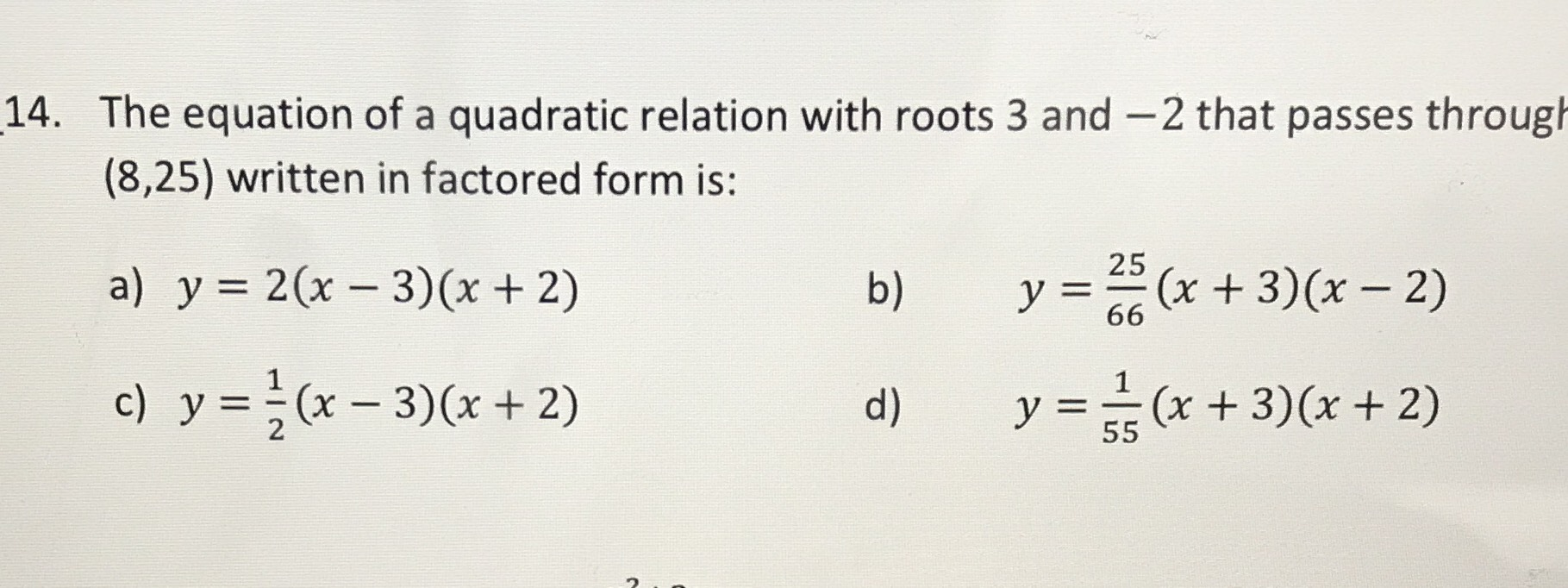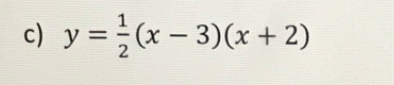### ¿Todavía tienes preguntas de matemáticas?

Pregunte a nuestros tutores expertos
Algebra
Pregunta14. The equation of a quadratic relation with roots $$3$$ and $$- 2$$ that passes through

$$( 8,25 )$$ written in factored form is:

a) $$y = 2 ( x - 3 ) ( x + 2 )$$ b) $$y = \frac { 25 } { 66 } ( x + 3 ) ( x - 2 )$$

c) $$y = \frac { 1 } { 2 } ( x - 3 ) ( x + 2 )$$ d) $$y = \frac { 1 } { 55 } ( x + 3 ) ( x + 2 )$$